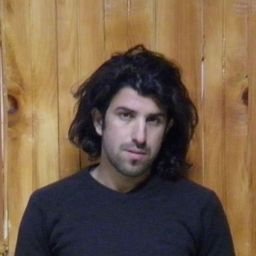### Discussion

You must be signed in to discuss.
##### Top Physics 101 Mechanics Educators##### Christina K.

Rutgers, The State University of New Jersey##### Andy C.

University of Michigan - Ann ArborLectures

Join Bootcamp

### Video Transcript

But if the given problem the angular speed is given by the tea divided by, um, are so then a BT will be 98 times of 0.447 Divided by, um, you're a 0.742 times to pie to pipe this kind of words are our omega into revolutions per second. That is 9.4 revolutions for a second Badri we confine the also who we called omega f squarely by by Omega Hi square. Well, I got to delta later. So many guys, they're also just find 9.4. He's only got if square um Omega f squaring minus zero divided by two times. So one revolution here Delta theta. This gives us off 44.2 revolutions per second square. These are awful. Then, from here we can find a r with a lot already likes emotion will be re t squared, divided by t. So here we have a 98 times, 0.447 all square divided by time. We have 01742 This gives us 2.59 times tented power. Three meter per second square is already a solution um, then I were credential. Exploration is are all thought. So is there a 0.7 for two dimes? 44.2 times to buy. This gives us 26 meter or second square eso This was about to be here, um, in boxy in the radial direction, off least point the hands support the weight of the bowl and also supplies. They see. So are centripetal acceleration. Then we can right there far more people to radio force with the mg. Oh, so and my radio exploration that isn't bolted in G plus they are so substituting values here 0.19 eighties our mass times 9.8 g plus 2.59 times 10 to the power three. Well, gives us here really enforce off 515 Newton in the tangential direction. Their hands supply only that initial insulation so we can write to me. F t is equal to m A t oh, substituting value 0.198 times two or six. Excuse us 40 40 Point it NewtonWesleyan University
##### Top Physics 101 Mechanics Educators##### Christina K.

Rutgers, The State University of New Jersey##### Andy C.

University of Michigan - Ann ArborLectures

Join Bootcamp